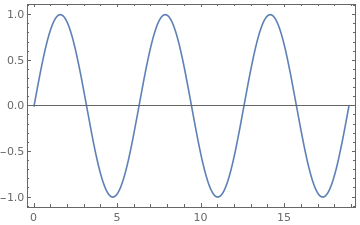## Background

I have been wanting to find a workflow which allows me to bypass writing a lot of TeX by hand for a while now. To that end I looked into using a computer algebra system (CAS). Naturally, my first choice was the FOSS Maxima (also because it uses Lisp under the hood). However, for all the reasons listed here, relating to its accuracy, which have not been fixed even though the post was over 5 years ago, I ended up having to go with the closed source Mathematica.

## Packages

Support for Mathematica in modern orgmode is mainly through the use of ob-mathematica, which is the official org-babel extension (from contrib) for working with Mathematica. However, ob-mathematica relies on the now-defunct mma package for font-locking, which is less than ideal. Thankfully, there exists the excellent wolfram-mode package which happens to be in MELPA as well. Finally, since the default return type of a mathematica block is an input-string meant to be used in another mathematica block, which is not useful when we work with org-babel, we will use the excellent mash.pl utility from here, as suggested by the ob-mathematica package to sanitize our output and set a unifying path.

So to recap, use your favorite manager to get:

• ob-mathematica (in contrib)
• wolfram-mode (MELPA)
• mash.pl (from here)1

After obtaining the packages, the configuration is then simply2:

 1;; Load mathematica from contrib
4                                     '((mathematica . t))
5                                     ))
6;; Sanitize output and deal with paths
7(setq org-babel-mathematica-command "~/.local/bin/mash")
8;; Font-locking
9(add-to-list 'org-src-lang-modes '("mathematica" . wolfram))
10;; For wolfram-mode
11(setq mathematica-command-line "~/.local/bin/mash")


## Results

### LaTeX

Now we are in a position to simply evaluate content with font-locking. We will test our set up with an example lifted from the ob-mathematica source-code.

A table
14
24
36
48
70
1(1+Transpose@x) // TeXForm


Where our header-line (with #+begin_src) is:

1mathematica :var x=example-table :results latex


### Sanity Checks

We can also test the example from the blog post earlier to test basic mathematical sanity.

1Limit[Log[b - a + I eta], eta -> 0, Direction -> -1,Assumptions -> {a > 0, b > 0, a > b}]
2TeXForm[Limit[Log[b - a + I eta], eta -> 0, Direction -> 1,Assumptions -> {a > 0, b > 0, a > b}]]


$$(I*Pi + Log[a - b])*\log (a-b)-i \pi$$

### Inline Math

Note that we can now also write fractions, integrals and other cumbersome TeX objects a lot faster with this syntax, like $$\frac{x^3}{3}$$. Where we are using the following snippet:

1src_mathematica[:exports none :results raw]{Integrate[x^2,x] // TeXForm}


### Plots

For plots, the standard orgmode rules apply, that is, we have to export to a file and return the name through our code snippet. Consider:

1p=Plot[Sin[x], {x, 0, 6 Pi},Frame->True];
2Export["images/sine.png",p];
3Print["images/sine.png"]Figure 1: An exported Mathematica image

Where we have used mathematica :results file as our header line.

1. As noted in the comments, it is nicer to rename mash.pl to mash ↩︎